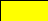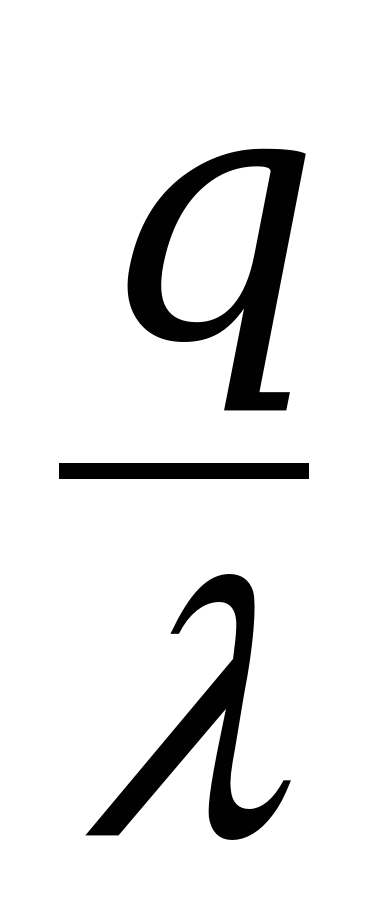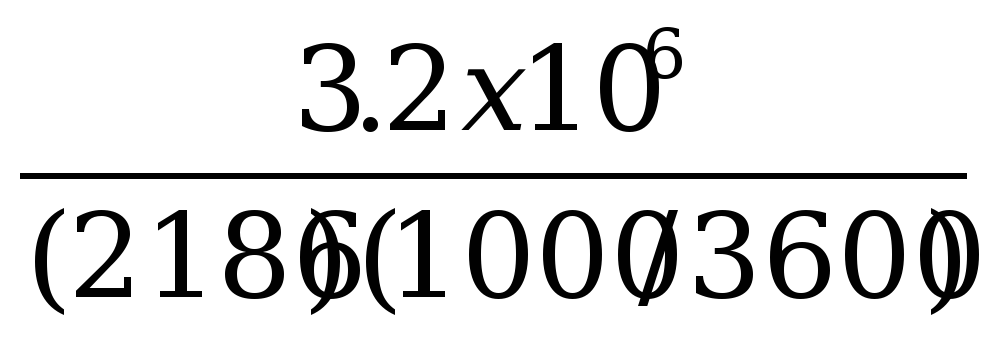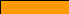# Contoh Soal Evaporasi 7 Sd 8.4-9.troughput Of A

8.4-9.Troughput of a single-effect evaporator. An evaporator is concentrating F kg/h at 311 K of a 20 wt % solution of NaOH to 50%. The saturated steam used for heating is at 399.3 K. the preesure in the vapor space of the evaporator is 13.3 Kpa absolute. The overall coefficient is 1420 W/m2.K and the area is 86.4 m2. Calculate the feed rate of the evaporator.

Solution :

• The heat capacity of the feed is assumed to be CpF = 4.14 kJ/kg.K

• Assumed boiling point of the dilute to be of the water at 101.32 KPa, T1 = 373.2 K (1000C) as the datum temperature.

• Hv latent heat of the water at 373.2 is 2257 kJ/kg base on steam table at appendix A.2

• Latent heat saturation temperature Ts = 399.3 K is 2186 kJ/kg.

• hL = 0, because its the datum of 3732.2 K

F.XF = L.XLF ( 0.2 ) = L (0.5 )

0.4 F = L

q = U A T

= 1420 ( 86.4 ) ( 399.3 373.2)

= 3.2 x 106

q= S S === 5269.89

F . hf + S . = L .hL + V . HV

F . ( 4.14 ) ( 311 373.2 ) + ( 5269.89 ) ( 2186 ) = L (0) + 0.4 F ( 2257)

-257.5 F + 1.152 x 107 = 902.8 F

1160.3 F = 1.152 x 107

F = 9072 kg/h